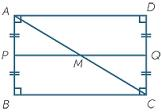# Calculate 5809

In the ABCD rectangle, the AB side is 16 cm long, and the AC diagonal is 20 cm long. Calculate the perimeter and area of the rectangle.

o =  56 cm
S =  192 cm2

### Step-by-step explanation:Did you find an error or inaccuracy? Feel free to write us. Thank you!

Tips for related online calculators
The Pythagorean theorem is the base for the right triangle calculator.